# How to calculate Distance in Python and Pandas using Scipy spatial and distance functions

Working with Geo data is really fun and exciting especially when you clean up all the data and loaded it to a dataframe or to an array. The real works starts when you have to find distances between two coordinates or cities and generate a distance matrix to find out distance of each city from other.

We will discuss in details about some performance oriented way to find the distances and what are the tools available to achieve that without much hassle.

In this post we will see how to find distance between two geo-coordinates using scipy and numpy vectorize methods

## Distance Matrix

As per wiki definition

In mathematics, computer science and especially graph theory, a distance matrix is a square matrix containing the distances, taken pairwise, between the elements of a set. If there are N elements, this matrix will have size N×N. In graph-theoretic applications the elements are more often referred to as points, nodes or vertices

Here is an example, A distance matrix showing distance of each of these Indian cities between each other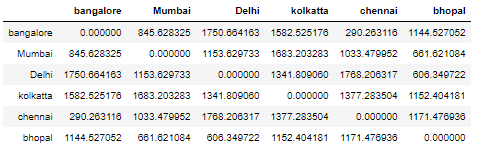## Haversine Distance Metrics using Scipy Distance Metrics Class

### Create a Dataframe

Let’s create a dataframe of 6 Indian cities with their respective Latitude/Longitude

``````from sklearn.neighbors import DistanceMetric
import pandas as pd
import numpy as np

cities_df = pd.DataFrame({'city':['bangalore','Mumbai','Delhi','kolkatta','chennai','bhopal'],
'lat':[12.9716,19.076,28.7041,22.5726,13.0827,23.2599],
'lon':[77.5946,72.877,77.1025,88.639,80.2707,77.4126],
})
``````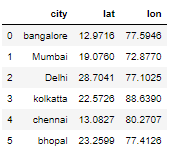### Convert the Lat/Long degress in Radians

In this step we will convert eh Lat/Long values in degrees to radians because most of the scipy distance metrics functions takes Lat/Long input as radians

``````cities_df['lat'] = np.radians(cities_df['lat'])
``````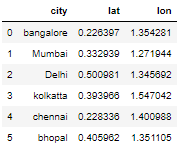### Scipy get_metrics()

Scipy has a distance metrics class to find out the fast distance metrics. You can access the following metrics as shown in the image below using the get_metrics() method of this class and find the distance between using the two points

Here is the table from the original scipy documentation :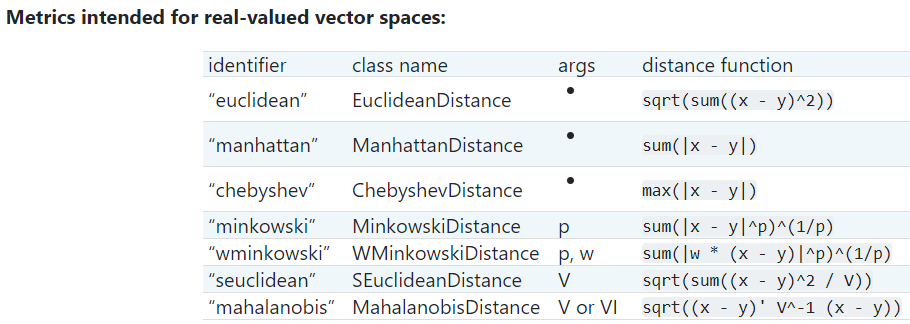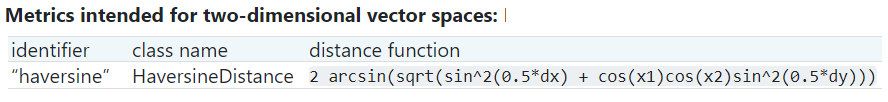Please check the documentation for other metrics to be use for other vector spaces

``````dist = DistanceMetric.get_metric('haversine')
``````

### Scipy Pairwise()

We have created a dist object with haversine metrics above and now we will use pairwise() function to calculate the haversine distance between each of the element with each other in this array

pairwise() accepts a 2D matrix in the form of [latitude,longitude] in radians and computes the distance matrix as output in radians too.

Input:

Input to pairwise() function is numpy.ndarray. So we have created a 2D matrix containing the Lat/Long of all the cities in the above dataframe

``````cities_df[['lat','lon']].to_numpy()

array([[12.9716, 77.5946],
[19.076 , 72.877 ],
[28.7041, 77.1025],
[22.5726, 88.639 ],
[13.0827, 80.2707],
[23.2599, 77.4126]])
``````

We will pass this ndarray in pairwise() function which returns the ouput as ndarray too

`````` dist.pairwise(cities_df [['lat','lon']].to_numpy())*6373
``````

Output:

Final Output of pairwise function is a numpy matrix which we will convert to a dataframe to view the results with City labels and as a distance matrix

Considering earth spherical radius as 6373 in kms, Multiply the result with 6373 to get the distance in KMS. For miles multiply by 3798

``````dist.pairwise(cities_df[['lat','lon']].to_numpy())*6373

array([[   0. ,  845.62832501, 1750.66416275, 1582.52517566,          290.26311647, 1144.52705214],
[ 845.62832501,    0. , 1153.62973323, 1683.20328341,         1033.47995206,  661.62108356],
[1750.66416275, 1153.62973323,    0. , 1341.80906015,         1768.20631663,  606.34972183],
[1582.52517566, 1683.20328341, 1341.80906015,    0. ,         1377.28350373, 1152.40418062],
[ 290.26311647, 1033.47995206, 1768.20631663, 1377.28350373,            0. , 1171.47693568],
[1144.52705214,  661.62108356,  606.34972183, 1152.40418062,         1171.47693568,    0. ]])
``````

### Create Dataframe of Distance Matrix

From the above output ndarray we will create a dataframe of distance matrix which will showcase distance of each of these cities from each other

So the index of this dataframe is the list of city and the columns are also the same city

Now if you look at the row and cell of any of the city it will show the distance between them

`````` pd.DataFrame(dist.pairwise(cities_df[['lat','lon']].to_numpy())*6373,  columns=cities_df.city.unique(), index=cities_df.city.unique())
``````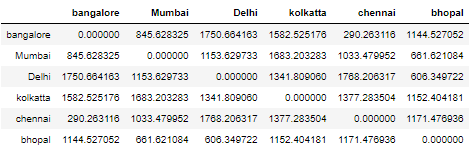## Euclidean Distance Metrics using Scipy Spatial pdist function

Scipy spatial distance class is used to find distance matrix using vectors stored in a rectangular array

We will check pdist function to find pairwise distance between observations in n-Dimensional space

Here is the simple calling format:

Y = pdist(X, ’euclidean’)

We will use the same dataframe which we used above to find the distance matrix using scipy spatial pdist function

pd.DataFrame(squareform(pdist(cities_df.iloc[:, 1:])), columns=cities_df.city.unique(), index=cities_df.city.unique())

We are using square form which is another function to convert vector-form distance vector to a square-form distance matrix, and vice-versa

Here also we convert all the Lat/long from degrees to radians and the output type is same numpy.ndarray

## Numpy Vectorize approach to calculate haversine distance between two points

For this we have to first define a vectorized function, which takes a nested sequence of objects or numpy arrays as inputs and returns a single numpy array or a tuple of numpy arrays

### Haversine Vectorize Function

Let’s create a haversine function using numpy

``````import numpy as np

def haversine_vectorize(lon1, lat1, lon2, lat2):

lon1, lat1, lon2, lat2 = map(np.radians, [lon1, lat1, lon2, lat2])

newlon = lon2 - lon1
newlat = lat2 - lat1

haver_formula = np.sin(newlat/2.0)**2 + np.cos(lat1) * np.cos(lat2) * np.sin(newlon/2.0)**2

dist = 2 * np.arcsin(np.sqrt(haver_formula ))
km = 6367 * dist #6367 for distance in KM for miles use 3958
return km
``````

Now here we need two sets of lat and long because we are trying to calculate the distance between two cities or points

### Dataframe with Orign and Destination Lat/Long

Let’s create another dataframe with Origin and destination Lat/Long columns

``````orig_dest_df = pd.DataFrame({
'origin_city':['Bangalore','Mumbai','Delhi','Kolkatta','Chennai','Bhopal'],
'orig_lat':[12.9716,19.076,28.7041,22.5726,13.0827,23.2599],
'orig_lon':[77.5946,72.877,77.1025,88.639,80.2707,77.4126],
'dest_lat':[23.2599,12.9716,19.076,13.0827,28.7041,22.5726],
'dest_lon':[77.4126,77.5946,72.877,80.2707,77.1025,88.639],
'destination_city':['Bhopal','Bangalore','Mumbai','Chennai','Delhi','Kolkatta']
})
``````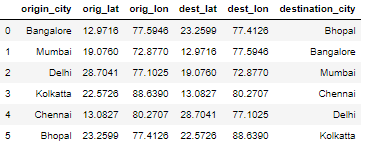### Calculate distance between origin and dest

Let’s calculate the haversine distance between origin and destination city using numpy vectorize haversine function

``````haversine_vectorize(orig_dest_df['orig_lon'],orig_dest_df['orig_lat'],orig_dest_df['dest_lon'],
orig_dest_df['dest_lat'])
``````
``````0    1143.449512
1     844.832190
2    1152.543623
3    1375.986830
4    1766.541600
5    1151.319225
dtype: float64
``````

### Add column to Dataframe using vectorize function

Let’s create a new column called haversine_dist and add to the original dataframe

``````orig_dest_df['haversine_dist'] = haversine_vectorize(orig_dest_df['orig_lon'],orig_dest_df['orig_lat'],orig_dest_df['dest_lon'],orig_dest_df['dest_lat'])
``````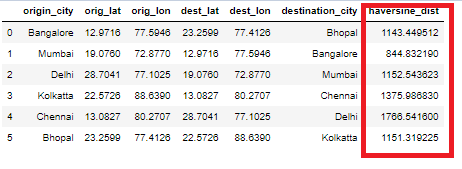It’s way faster than normal python looping and using the timeit function I can see the performance is really tremendous.

``````%%timeit
orig_dest_df['haversine_dist'] = haversine_vectorize(orig_dest_df['orig_lon'],orig_dest_df['orig_lat'],orig_dest_df['dest_lon'],orig_dest_df['dest_lat'])
``````

18.5 ms ± 4.49 ms per loop (mean ± std. dev. of 7 runs, 10 loops each)

We have a small dataset but for really large data in millions also it works fast with this vectorize approach

## Conclusion:

So far we have seen the different ways to calculate the pairwise distance and compute the distance matrix using Scipy’s spatial distance and Distance Metrics class.

Scipy Distance functions are a fast and easy to compute the distance matrix for a sequence of lat,long in the form of [long, lat] in a 2D array. The output is a numpy.ndarray and which can be imported in a pandas dataframe

Using numpy and vectorize function we have seen how to calculate the haversine distance between two points or geo coordinates really fast and without an explicit looping

Do you know any other methods or functions to calculate distance matrix between vectors ? Please write your comments and let us know

Tags:

Categories:

Updated: